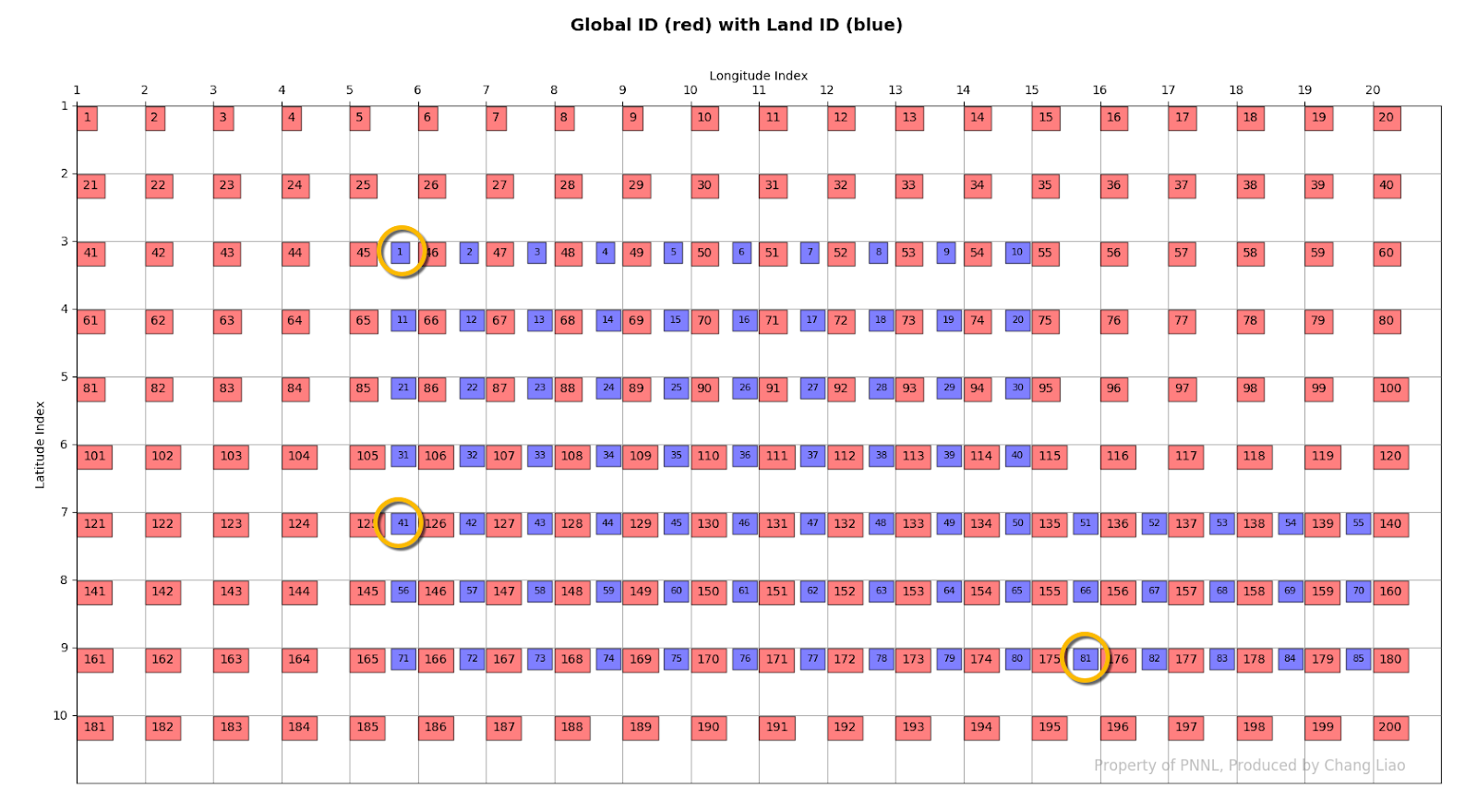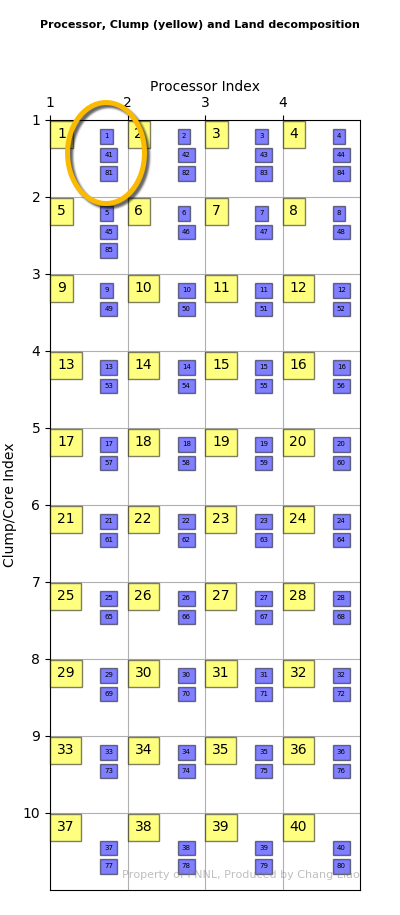### A visual decomposition of the land grid in the E3SM/CESM model

One of my recent development projects needs to exchange several variables between different components using the Common Infrastructure for Modeling the Earth (CIME) model. However, different components have different grid systems. Therefore, I have to get familiar with the grid system of these two component before assigning any variables.

One of them is the land grid used in Community Land Model (CLM)/ELM, which is the land component in the Energy Exascale Earth System Model (E3SM) model. Because CLM is also part of CESM, this post should also apply to the CESM land component.

In short, the land component distributes all the land units across multiple nodes/cores and each grid cell is run by clump.

Here I developed a small Python utility to illustrate the concept.

First we define a sample problem as follow:

 Variable Value Description npes 4 CPU/Node clump_pproc 10 Core per CPU nsegspc 2 Segment per clump nclumps 40 Core count lni 20 Longitude count lnj 10 Latitude count numg 85 Land count

Then we define the global grid and land mask:

Before you start to read the numbers, you need to understand the index system used in E3SM.
Even though Fortran is a column-major language, the E3SM (written in Fortran) stores the global grid using a row-major approach. As a result, global grid index starts from left to right in memory, same as the land mask (Figure 1).

Note that yellow square represent clump index, blue square represent land index, and red square represents global grid index.Figure 1. The global ID and land ID map.

Similar to global grid, clump/core index starts from left to right across node/processor. For example, clump 1 is located on the first core on processor 1 and clump 2 is located on the first core on processor 2.

Last, we distribute the land grid onto the clumps. All the land grids are allocated to clump in order. In the end, a clump may simulate multiple land grids which are far away in the spatial domain. For example, the clump 1 simulates land grid 1, 41 and 81 (circled in yellow in Figure 1 and 2).Figure 2. The processor, clump and land grid decomposition.

The global grid and land grid is connected through the “gdc2glo” variable, which stores what is the land grid index for each clump in order. For example, gdc2glo = 125 means that the 125th global grid is the “second” (index starting from 0) simulated land grid, which is land grid 41.

Let me know if you have any question.

### Spatial datasets operations: mask raster using region of interest

Climate change related studies usually involve spatial datasets extraction from a larger domain.
In this article, I will briefly discuss some potential issues and solutions.

In the most common scenario, we need to extract a raster file using a polygon based shapefile. And I will focus as an example.

In a typical desktop application such as ArcMap or ENVI, this is usually done with a tool called clip or extract using mask or ROI.

Before any analysis can be done, it is the best practice to project all datasets into the same projection.

If you are lucky enough, you may find that the polygon you will use actually matches up with the raster grid perfectly. But it rarely happens unless you created the shapefile using "fishnet" or other approaches.

What if luck is not with you? The algorithm within these tool usually will make the best estimate of the value based on the location. The nearest re-sample, but not limited to, will be used to calculate the value. But what about the outp…

### Numerical simulation: ode/pde solver and spin-up

For Earth Science model development, I inevitably have to deal with ODE and PDE equations. I also have come across some discussion related to this topic, i.e.,

https://www.researchgate.net/post/What_does_one_mean_by_Model_Spin_Up_Time

In an attempt to answer this question, as well as redefine the problem I am dealing with, I decided to organize some materials to illustrate our current state on this topic.

Models are essentially equations. In Earth Science, these equations are usually ODE or PDE. So I want to discuss this from a mathematical perspective.

Ideally, we want to solve these ODE/PDE with initial condition (IC) and boundary condition (BC) using various numerical methods.
https://en.wikipedia.org/wiki/Initial_value_problem
https://en.wikipedia.org/wiki/Boundary_value_problem

Because of the nature of geology, everything is similar to its neighbors. So we can construct a system of equations which may have multiple equation for each single grid cell. Now we have an array of equation…

### Watershed Delineation On A Hexagonal Mesh Grid: Part A

One of our recent publications is "Watershed Delineation On A Hexagonal Mesh Grid" published on Environmental Modeling and Software (link).
Here I want to provide some behind the scene details of this study.

(The figures are high resolution, you might need to zoom in to view.)

First, I'd like to introduce the motivation of this work. Many of us including me have done lots of watershed/catchment hydrology modeling. For example, one of my recent publications is a three-dimensional carbon-water cycle modeling work (link), which uses lots of watershed hydrology algorithms.
In principle, watershed hydrology should be applied to large spatial domain, even global scale. But why no one is doing it?  I will use the popular USDA SWAT model as an example. Why no one is setting up a SWAT model globally?
There are several reasons we cannot use SWAT at global scale: We cannot produce a global DEM with a desired map projection. SWAT model relies on stream network, which depends on DEM.…